# RMO 2016 Delhi Region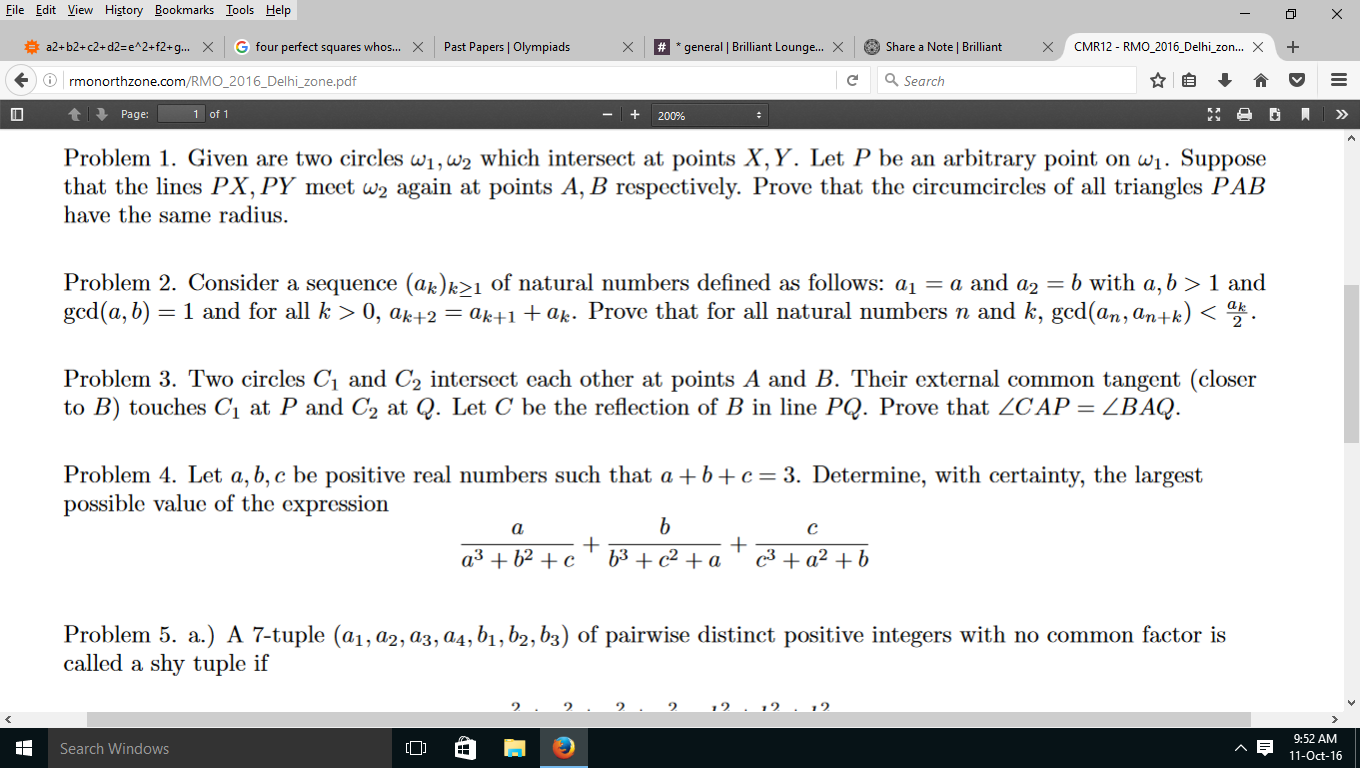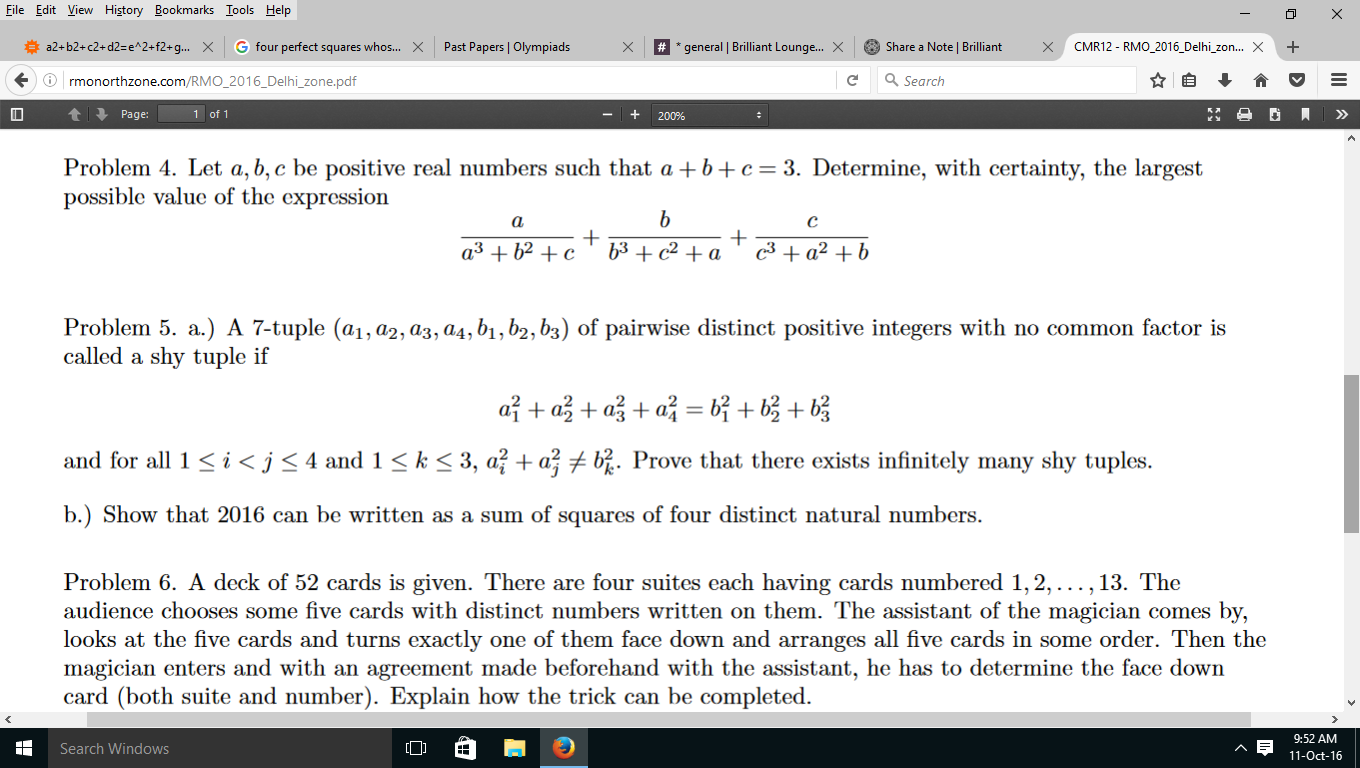Here is the paper of RMO 2016 Delhi.Pls post answers and solutions to all questions. Also tell ur marks and estimated cut off Thanks!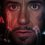Note by Kaustubh Miglani
4 years, 6 months ago

This discussion board is a place to discuss our Daily Challenges and the math and science related to those challenges. Explanations are more than just a solution — they should explain the steps and thinking strategies that you used to obtain the solution. Comments should further the discussion of math and science.

When posting on Brilliant:

• Use the emojis to react to an explanation, whether you're congratulating a job well done , or just really confused .
• Ask specific questions about the challenge or the steps in somebody's explanation. Well-posed questions can add a lot to the discussion, but posting "I don't understand!" doesn't help anyone.
• Try to contribute something new to the discussion, whether it is an extension, generalization or other idea related to the challenge.
• Stay on topic — we're all here to learn more about math and science, not to hear about your favorite get-rich-quick scheme or current world events.

MarkdownAppears as
*italics* or _italics_ italics
**bold** or __bold__ bold
- bulleted- list
• bulleted
• list
1. numbered2. list
1. numbered
2. list
Note: you must add a full line of space before and after lists for them to show up correctly
paragraph 1paragraph 2

paragraph 1

paragraph 2

[example link](https://brilliant.org)example link
> This is a quote
This is a quote
    # I indented these lines
# 4 spaces, and now they show
# up as a code block.

print "hello world"
# I indented these lines
# 4 spaces, and now they show
# up as a code block.

print "hello world"
MathAppears as
Remember to wrap math in $$ ... $$ or $ ... $ to ensure proper formatting.
2 \times 3 $2 \times 3$
2^{34} $2^{34}$
a_{i-1} $a_{i-1}$
\frac{2}{3} $\frac{2}{3}$
\sqrt{2} $\sqrt{2}$
\sum_{i=1}^3 $\sum_{i=1}^3$
\sin \theta $\sin \theta$
\boxed{123} $\boxed{123}$

## Comments

Sort by:

Top Newest

Please explain the statement above the concluding statement

- 3 years, 2 months ago

Log in to reply

Problem 4

By the Cauchy-Schwarz inequality, $\left(\dfrac{1}{a}+1+c\right)(a^{3}+b^{2}+c) \geq (a+b+c)^{2}=9$

$\implies (ac+a+1)(a^{3}+b^{2}+c) \geq 9a$

Thus, we get

$f(a,b,c)=\quad \sum {\dfrac{(ac+a+1)a}{(ac+a+1)({a}^{3}+{b}^{2}+{c})}} \leq \quad \sum \dfrac{a(ac+a+1)}{9a}$

$\implies f(a,b,c) \leq \dfrac{1}{9}[(ac+a+1)+(ba+b+1)+(cb+c+1)]$

$=\dfrac{1}{9}[(a+b+c)+(1+1+1)+(ab+bc+ca)] \leq \dfrac{1}{9}[3+3+3]=\boxed{1}$

Here we used the identity $(a+b+c)^{2} \geq 3(ab+bc+ca)$ to get $ab+bc+ca \leq 3$

- 3 years, 7 months ago

Log in to reply

Need a solution for q 2 and 6

- 3 years, 9 months ago

Log in to reply

Can anyone post complete solution to 2nd and last problem?

- 3 years, 9 months ago

Log in to reply

Problem 5 1)its the easiest- use the identity ${ (x+y+z) }^{ 2 }+{ (x+y-z) }^{ 2 }+{ (x-y+z) }^{ 2 }+{ (-x+y+z) }^{ 2 }={ (2x) }^{ 2 }+{ (2y) }^{ 2 }+{ (2z) }^{ 2 }$ which gives infinite solutions in integers x,y,z.u can check it satisfis all other cond.s 2)solve this one other way round, assume 2016 = ${ (2x) }^{ 2 }+{ (2y) }^{ 2 }+{ (2z) }^{ 2 }$ then it implies 504 = ${ (x) }^{ 2 }+{ (y) }^{ 2 }+{ (z) }^{ 2 }$ by inspection x=22 y=4 z=6, then use the identity Problem 2 -we obtain nth term in terms of n,a,b then use strong induction.

- 3 years, 9 months ago

Log in to reply

the question says pairwise distinct positive integers with no common factor, doesn't that mean they're pairwise coprime?

- 3 years, 9 months ago

Log in to reply

The question mentions that they all don't have a factor in common, and that doesn't mean they are pairwise relatively prime. If x,y, and z are relatively prime, then we are done because then the gcd of these 7 terms would be 1 :)

- 3 years, 8 months ago

Log in to reply

oh, thank you i didn't understand the question properly then

- 3 years, 7 months ago

Log in to reply

Diagram to problem $3$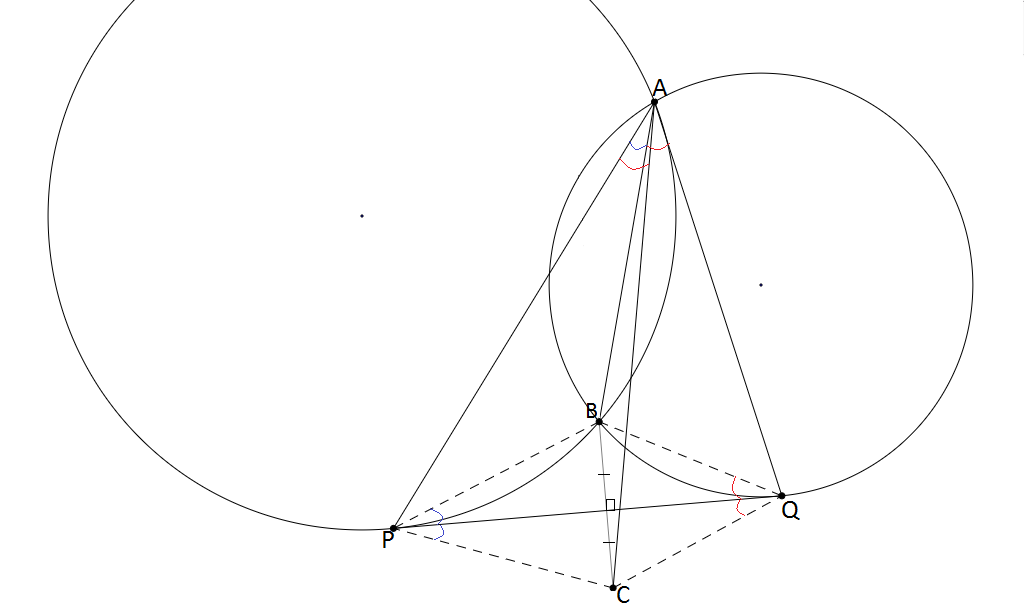Log in to reply

Instead,use A-Humpty point lemma, which proves it without anything.

- 3 years, 9 months ago

Log in to reply

Yup, and then repeat the same procedure to prove the lemma in examination.

- 3 years, 9 months ago

Log in to reply

So in exam, to do any inequality, we can use only upto am-gm? not even cauchy? or can we not use even weighted am-gm?

- 3 years, 9 months ago

Log in to reply

You can use AM-GM and weighted AM-GM because at times some problems are not easy to prove without weighted AM-GM{RMO 2012 paper1 p3}. Use Cauchy-Schwarz too because it is of the basic inequalities. If you use a lemma like the Titu's lemma, then better prove it for RMO, I guess no need to prove that in INMO, not IMO definitely!

- 3 years, 7 months ago

Log in to reply

Hm... All those words go above me. Humpty-Dumpty theorem (Both different) are well-known. Although not that standard to be used directly in exams. I have seen guys proving each and every lemma, all for the sake of marks only/.

- 3 years, 9 months ago

Log in to reply

so by standard textbooks, do they mean ncert?

- 3 years, 9 months ago

Log in to reply

What? No.......

- 3 years, 9 months ago

Log in to reply

Solution to $3$

Const:-- Join $BP$ , $BQ$ , $CP$ & $CQ.$

Clearly, Using Alternate Segment Theorem , we get:$\color{#D61F06}{\angle BAQ = \angle BQP}$ and $\color{#3D99F6}{\angle BAP = \angle BPQ}$.--- $$

Also Using the isosceles triangle property, we get :: $\color{#D61F06}{\angle PQC = \angle BQP}$ & $\color{#3D99F6}{\angle QPC = \angle BPQ}$-----$$

Thus from $eq^{n}$ $$ and $$ we get: $\color{#D61F06}{\angle BAQ = \angle PQC}$ & $\color{#3D99F6}{\angle BAP = \angle QPC}$.

Adding these two results we get: $\angle BAQ + \angle BAP$ = $\angle PQC + \angle QPC$

Thus $\angle PCQ$ = $180^{\circ}-[\angle PQC + \angle QPC]$ = $180^{\circ}-[\angle BAQ + \angle BAP]$

Therefore, $\angle PCQ + PAQ$ = $180^{\circ}-[\angle BAQ + \angle BAP] + [\angle BAP + \angle BAQ]$ = $180^{\circ}$

=>$\quad APCQ$ is cyclic

=> $\angle PAC= \color{#D61F06}{\angle PQC = \angle BAQ}$

=> $\angle PAC = \angle BAQ$

Log in to reply

Diagram to problem 1.........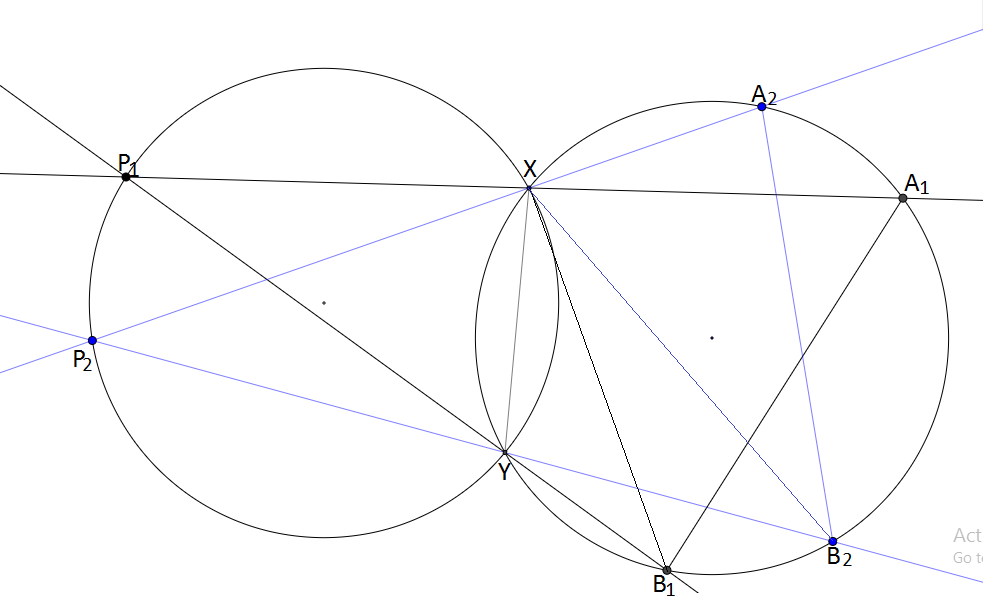Log in to reply

Pls do provide the solution of the last problem

- 3 years, 7 months ago

Log in to reply

Can anyone give complete solution of 2 and 6

- 3 years, 9 months ago

Log in to reply

@Azimuddin Sheikh Idk latex so cant post soln to 2

- 3 years, 9 months ago

Log in to reply

@Azimuddin Sheikh At least two cards have same suit let P1, P2 has same suit then the assistant will turn down one of P1, P2 (say P1) and arrange P2, P3, P4, P5 in such a way that P2 takes first position so the magician know that the card turned down and the card having first position have same suit. This will help him to identify suit of P1 now there are 6 ways to arrange P3 P4 P5 as LMH, LHM, MLH, MHL, HLM, HML (low number, middle number, high number) If difference of numbers obtained on P1 and P2 is 6 then turn down the higher one and give first position to lower one. Let us assume P1 has number k and P2 has no k + 1, k + 2, k + 3, k + 4, k + 5 or k + 6. If P2 has k + 1 then show LMH. If P2 has k + 2 then show LHM and soon. If difference is > 6 then turn down lower one and give first position to higher one and add 1,2,3,4,5,6 for arrangements LMH, LHM, MLH, MHL, HLM, HML in the higher number for example adding 4 to 11 indicates 2.

- 3 years, 9 months ago

Log in to reply

The last combinatorics question is more or less the same as the first question in the chapter combinatorics from the book 'The art and craft of problem-solving'

- 3 years, 8 months ago

Log in to reply

Solution to Problem 1. Let $P_{1}$ and $P_{2}$ be the two points on $\odot\omega_{1}$ as $P$ varies across the circumference of $\odot \omega_{1}.$ Similarly, let $P_{1}X$ and $P_{1}Y$ meet $\odot\omega_{2}$ at $A_{1}$ , $B_{1}$ and $P_{2}X$ and $P_{2}Y$ meet $\odot\omega2$ at $A_{2}$ , $B_{2}$ respectively.

Clearly , $\angle XP_{1}Y = \angle XP_{2}Y$ and $\angle XB_{1}Y = \angle XB_{2}Y$

Adding these two $eq^{n}$ we get:: $\angle A_{1}XB_{1} = \angle A_{2}XB_{2}$ => $A_{1}B_{1} = A_{2}B_{2}.$

Also, $\Delta XP_{1}Y \sim \Delta A_{1}P_{1}B_{1}$ => $\dfrac{Radius XP_{1}Y}{Radius A_{1}P_{1}B_{1}}$ = $\dfrac{XY}{A_{1}B_{1}}$ ........[ $1$ ]

Similarly, $\Delta XP_{2}Y \sim \Delta A_{2}P_{2}B_{2}$

=> $\dfrac{Radius XP_{2}Y}{Radius A_{2}P_{2}B_{2}}$ = $\dfrac{XY}{A_{2}B_{2}}$

=> $\dfrac{Radius XP_{1}Y}{Radius A_{2}P_{2}B_{2}}$ = $\dfrac{XY}{A_{1}B_{1}}$ ........[ $2$ ]

[Because $\Delta XP_{1}Y$ and $\triangle XP_{2}Y$ have the same circumcircle. & $A_{1}B_{1} = A_{2}B_{2}$]

From $eq^{n}$ $$ and $$ we get that :: Radius $A_{1}P_{1}B_{1}$ = Radius $A_{2}P_{2}B_{2}.$

$K.I.P.K.I.G$

Log in to replyProblem 1

We can see that as we move the point $P$ on the circumference of the circle$[$excluding $X$ and $Y],$the $\angle XPY=\angle XP_1Y$ remains constant.So this shows that $AB=A_1B_1.$Now we use extended sin rule to complete the problem.
Let the circum-radius of $\triangle PAB$ be $R$ and $\triangle P_1A_1B_1$ be $R_1.$
In $\triangle PAB, \frac{AB}{sin\angle P}=2R$ and in $\triangle P_1A_1B_1,\frac{A_1B_1}{sin\angle P_1}=\frac{AB}{sin\angle P}=2R_1.$ Therefore $2R=2R_1\Rightarrow\boxed {R=R_1}.$Hence Proved.

- 4 years, 6 months ago

Log in to reply

Hey can u explain in detail why A1B1=AB

- 4 years, 3 months ago

Log in to reply

Because angle subtended by both of these chords at centre are equal. angle A1 X A =P X P1=P Y P1=B Y B1

- 4 years, 3 months ago

Log in to reply

Which class are u in and which school?

- 4 years, 3 months ago

Log in to reply

I m in class 10 And from bbps dw. But ur profile says u live in noida

- 4 years, 3 months ago

Log in to reply

I am graduating from IIT Bombay. What is ur favourite college bro?

- 4 years, 3 months ago

Log in to reply

IIT Is the one Bombay ,Perhaps

- 4 years, 3 months ago

Log in to reply

Prefered Jee rank?mine was 17

- 4 years, 3 months ago

Log in to reply

Do u remember books you used for iit preparation.I am an iit aspirant

- 3 years, 10 months ago

Log in to reply

hcv,Bmsharma,resnick,irodov,krotov,kolenkow -Phy atkins,rc mukerje,bahadur,jd lee-Chm sl loney,hallknight,mostly tatamcgraw buks and tons of practise question papers here at allen.u can download them online also.Ncert is must .Hope it helps.

- 3 years, 9 months ago

Log in to reply

Hi Where Can I find some good material for RMO this year? Last time I failed miserably :'( could solve just one... Thanks :)

- 3 years, 10 months ago

Log in to reply

first solve all previous year question papers, then use brilliant if ur aspiration is limited to rmo not inmo or selection camp P.S. i did 4 at my time

- 3 years, 9 months ago

Log in to reply

would u like to join my RMO/INMO group. if yes then give ur email.

- 3 years, 10 months ago

Log in to reply

My email is adityak1135@gmail.com I know that this is a really late reply but I didn't know about it earlier ;)

- 3 years, 7 months ago

Log in to reply

My email yashmehan@gmail.com Sorry for the late reply I do not often open up Brilliant Thanks!!!

- 3 years, 7 months ago

Log in to reply

is dis question addressed to me?

- 3 years, 9 months ago

Log in to reply

Yeah he is good.Invite him

- 3 years, 10 months ago

Log in to reply

what about u?

- 3 years, 10 months ago

Log in to reply

what about me?

- 3 years, 10 months ago

Log in to reply

wont u join our group?

- 3 years, 10 months ago

Log in to reply

he is already in there @ayush rai

- 3 years, 9 months ago

Log in to reply

nice solution

- 4 years, 6 months ago

Log in to reply

×

Problem Loading...

Note Loading...

Set Loading...